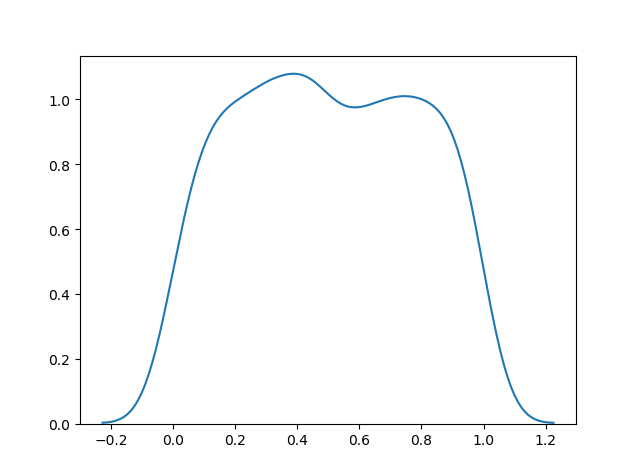# Uniform Distribution

## Uniform Distribution

Used to describe probability where every event has equal chances of occuring.

E.g. Generation of random numbers.

It has three parameters:

`a` - lower bound - default 0 .0.

`b` - upper bound - default 1.0.

`size` - The shape of the returned array.

### Example

Create a 2x3 uniform distribution sample:

from numpy import random

x = random.uniform(size=(2, 3))

print(x)
Try it Yourself »

## Visualization of Uniform Distribution

### Example

from numpy import random
import matplotlib.pyplot as plt
import seaborn as sns

sns.distplot(random.uniform(size=1000), hist=False)

plt.show()

### ResultTry it Yourself »

W3Schools is optimized for learning and training. Examples might be simplified to improve reading and learning. Tutorials, references, and examples are constantly reviewed to avoid errors, but we cannot warrant full correctness of all content. While using W3Schools, you agree to have read and accepted our terms of use, cookie and privacy policy.